StatLect

Jensen's inequality

Jensens's inequality concerns the expected value of convex and concave transformations of a random variable.Statement

The following is a formal statement of the inequality.

Proposition Letbe an integrable random variable. Letbe a convex function such thatis also integrable. Then, the following inequality, called Jensen's inequality, holds:Proof

A functionis convex if, for any pointthe graph oflies entirely above its tangent at the point:whereis the slope of the tangent. Settingand, the inequality becomesTaking the expected value of both sides of the inequality and using the fact that the expected value operator preserves inequalities, we obtain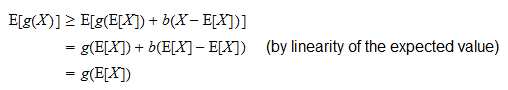If the functionis strictly convex andis not almost surely constant, then we have a strict inequality:Proof

A functionis strictly convex if, for any pointthe graph oflies entirely above its tangent at the point(and stricly so for points different from):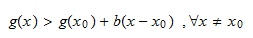whereis the slope of the tangent. Settingand, the inequality becomes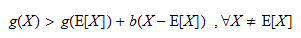and, of course,when. Taking the expected value of both sides of the inequality and using the fact that the expected value operator preserves inequalities, we obtainwhere the first inequality is strict because we have assumed thatis not almost surely constant and therefore the eventdoes not have probability.

If the functionis concave, thenProof

Ifis concave, thenis convex and by Jensen's inequality:Multiplying both sides byand using the linearity of the expected value we obtain the result.

If the functionis strictly concave andis not almost surely constant, thenProof

Similar to previous proof.

Example

Suppose a strictly positive random variablehas expected valueand it is not constant with probability one. What can we say about the expected value of, by using Jensen's inequality?

The natural logarithm is a strictly concave function, because its second derivativeis strictly negative on its domain of definition.

As a consequence, by Jensen's inequality, we have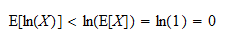Therefore,has a strictly negative expected value.

Exercise 1

Letbe a strictly positive random variable, such thatWhat can you infer, using Jensen's inequality, about the following expected value:Solution

The functionhas first derivativeand second derivativeThe second derivative is strictly negative on the domain of definition of the function. Therefore, the function is strictly concave. Furthermore,is not almost surely constant, because it has strictly positive variance. Hence, by Jensen's inequality: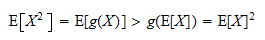The book

Most of the learning materials found on this website are now available in a traditional textbook format.

Glossary entries
Share